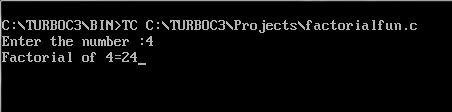UncleCoder.com

UncleCoder.com

Free programming examples and instructions

# C program to find factorial of a number using function

## C program to find factorial of a number

by Krishna

Posted on 27 Jul 2018 Category: c Views: 2209

C program to find factorial of a number

Below shows the C program to find factorial of a number using function.

``````#include <stdio.h>

long factorial(int);

int main()
{
int number;
long fact = 1;

printf("Enter a number :");
scanf("%d", &number);

printf("Factorial of %d = %ld\n", number, factorial(number));

return 0;
}

long factorial(int n)
{
int c;
long result = 1;

for (c = 1; c <= n; c++)
result = result * c;

return result;
}
``````

OUTPUT### Latest posts in c

##### C program to find largest among two numbers• View all

• View all

• View all

• View all

• View all